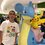# Multi-Pole Problem

So we all know the infamous Two-Pole Problem, which doesn't rely on the distances between the two poles. I have a situation where there are a n poles. Each pair of consecutive poles is used to create a Two-Pole situation, thus creating n-1 shorter poles. Using these, n-2 shorter poles are created in a similar fashion. This continues until there is one pole, which for reference I will call the "supporting pole". if $x_{1}, x_{2}, x_{3}, ..., x_{n}$ are the lengths of the starting poles, find a formula for the length of the supporting pole in a n-Pole Problem.Note by Tristan Shin
7 years, 3 months ago

This discussion board is a place to discuss our Daily Challenges and the math and science related to those challenges. Explanations are more than just a solution — they should explain the steps and thinking strategies that you used to obtain the solution. Comments should further the discussion of math and science.

When posting on Brilliant:

• Use the emojis to react to an explanation, whether you're congratulating a job well done , or just really confused .
• Ask specific questions about the challenge or the steps in somebody's explanation. Well-posed questions can add a lot to the discussion, but posting "I don't understand!" doesn't help anyone.
• Try to contribute something new to the discussion, whether it is an extension, generalization or other idea related to the challenge.

MarkdownAppears as
*italics* or _italics_ italics
**bold** or __bold__ bold
- bulleted- list
• bulleted
• list
1. numbered2. list
1. numbered
2. list
Note: you must add a full line of space before and after lists for them to show up correctly
paragraph 1paragraph 2

paragraph 1

paragraph 2

[example link](https://brilliant.org)example link
> This is a quote
This is a quote
    # I indented these lines
# 4 spaces, and now they show
# up as a code block.

print "hello world"
# I indented these lines
# 4 spaces, and now they show
# up as a code block.

print "hello world"
MathAppears as
Remember to wrap math in $$ ... $$ or $ ... $ to ensure proper formatting.
2 \times 3 $2 \times 3$
2^{34} $2^{34}$
a_{i-1} $a_{i-1}$
\frac{2}{3} $\frac{2}{3}$
\sqrt{2} $\sqrt{2}$
\sum_{i=1}^3 $\sum_{i=1}^3$
\sin \theta $\sin \theta$
\boxed{123} $\boxed{123}$

Sort by:

$\frac{1}{\sum_{i=1}^n\frac{1}{x_{i}}}$

- 7 years, 3 months ago

That's close. Try a few other cases that can disprove this(try 5 poles, for instance).

- 7 years, 3 months ago

- 7 years, 3 months ago

I don't know that problem (you said it's infamous then why not show it)

- 7 years, 3 months ago

For clarification, the Two-Pole Problem is where there are two poles of length a and b. Lines are drawn from the top of each to the bottom of the other. From the intersection of these two new lines, a vertical line segment parallel to the original poles is drawn. It turns out that the distance between the two poles is irrelevant. To solve this problem, try playing around with a few two-pole scenarios.

- 7 years, 3 months ago

I'll reveal this solution within the next week(April 21-April 25).

- 7 years, 3 months ago

I think now you should reveal the solution.....

- 7 years, 3 months ago

We use the formula $\dfrac{1}{x}+\dfrac{1}{y}=\dfrac{1}{z}$ where $x,y$ are the two poles and $z$ is the height of the middle pole. Solving for the supporting pole is pretty trivial, just plug 'n' chug.

- 7 years, 3 months ago

But is there a generic formula for the supporting pole, not just plugging it in?

- 7 years, 3 months ago